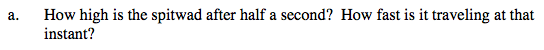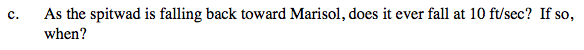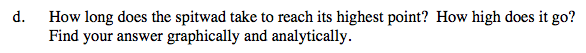### Home > CALC > Chapter 5 > Lesson 5.1.1 > Problem5-5

5-5.Use the equation, h(t), that you found in problem 5-1 to find the height of the spitwad after 2 seconds.

'How fast' could refer to velocity or speed. Be sure that you are clear in your answer.x(t) = 10v(t) = −10

$(\text{show steps})=\frac{37}{16}\text{ seconds}$The spitwad will be at its highest point at the moment when its velocity changes from positive to negative.